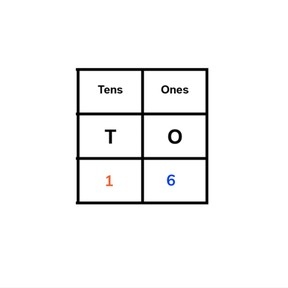Place value- numbers in charts to 20

# Place value- numbers in charts to 20

Place value- numbers in charts to 20

No account needed.8,000 schools use Gynzy92,000 teachers use Gynzy1,600,000 students use Gynzy

## General

Students learn to place numbers to 20 into a TO-chart. They learn which tens and ones make numbers to 20 and can place them in the chart.

-K.NBT.A.1

## Relevance

Discuss with students that it is important to know how numbers to 20 are put together so you can calculate with them later.

## Introduction

The interactive whiteboard shows a row of books and blocks with numbers. The students are asked to count forwards and backwards to fill in the missing numbers. Ask students to write down the numbers and then erase the covers to check their answers. Next place an amount of rulers (maximum 20) on the table in groups of ten and ask a student to come up and say how many there are. Do the same with some scissors and notebooks.

## Development

Show tens and ones on the interactive whiteboard. Explain that tens are a group of 10 and ones are the numbers 1 to 9. Show the MAB blocks on the interactive whiteboard and/or in class. Using the blocks, clarify the explanation of tens and ones. Next show the chart of tens and ones. Discuss an example that only has ones, 2. Next to the number is a TO(tens/ones)-chart with the tens and ones filled in. Say that when there are no tens, you fill in a 0 on the chart. Practice as a class, have students say what the tens and ones should be and complete the TO-chart. Next show a number that contains both tens and ones. Show the completed TO-chart and practice as a class with tens and ones. Students must indicate what are the tens and what are the ones of the given number. Practice a few exercises as a class. Most have visual support but the final example has only the number.

Check that students understand how numbers to 20 are put together and that they can complete a TO-chart by asking the following questions:
- What are tens? How do you know?
- What are ones? How do you know?
- Fill in a TO-chart for the number 17. What are the tens? ones?

## Guided Practice

Students first practice completing a TO-chart with visual support. Then they practice completing the chart with only the number.

## Closing

Discuss with students that it is important to know how numbers to 20 are put together and how to put them in a TO-chart so you can calculate with them later. Check that students understand what tens and ones are, and how to fill them into a TO-chart. To practice this again, students get to work in pairs. Both students write numbers (to 20) on a sheet of paper, and the other student must place the number into a TE- chart.

## Teaching Tip

Students who have difficulty with placing numbers into the TO-chart can be helped by visualizing the amounts with MAB blocks or manipulatives. Have them move the blocks of the number to the positions on the chart.

## Instruction materials

rulers, scissors, notebooks, MAB blocks

### The online teaching platform for interactive whiteboards and displays in schools

• Save time building lessons

• Manage the classroom more efficiently

• Increase student engagement